# 边缘检测(Edge Detection)

## 目标

• 图像的大部分语义信息和形状信息都可以编码在边缘上
• 理想:艺术家使用线条勾勒画(但艺术家也使用对象层次的知识)

• 表面形状的突变
• 深度方向的不连续
• 表面颜色的突变
• 光线阴影的不连续

## 边缘的特征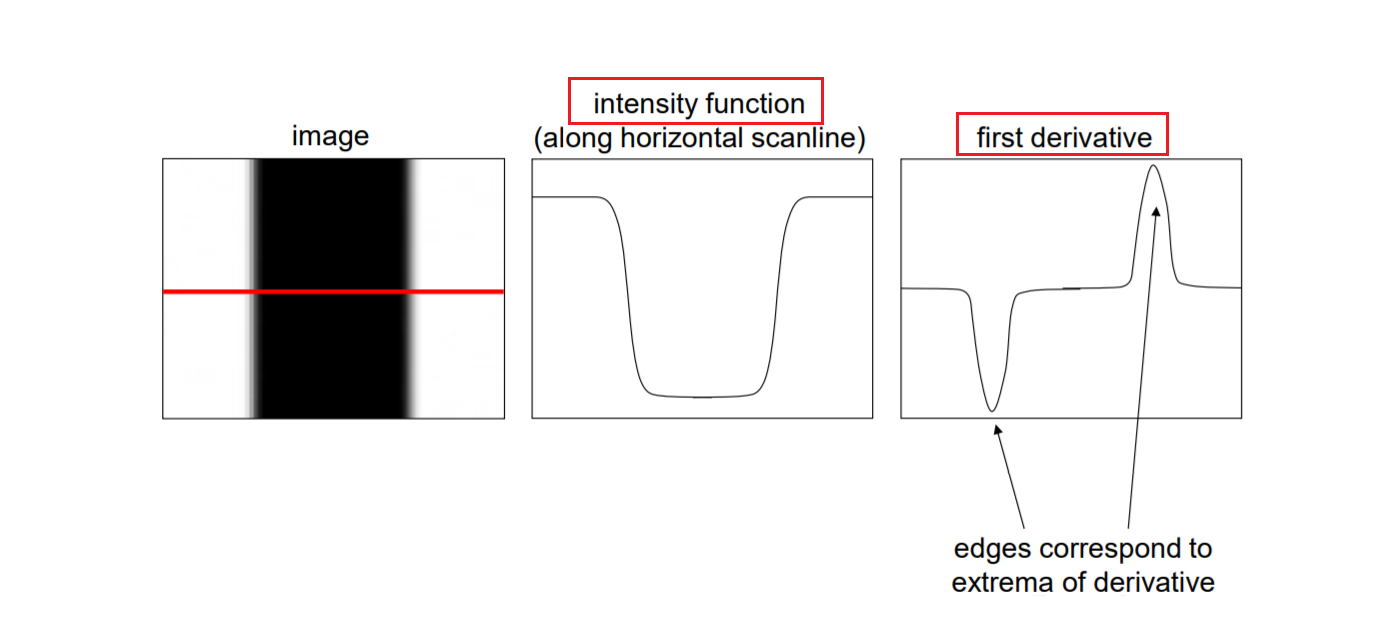• 偏导数公式：

$$\frac {\partial f(x,y)}{\partial x} = \lim_{\varepsilon \rightarrow 0} \frac{f(x+\varepsilon ,y)-f(x,y)}{\varepsilon}$$

• 在卷积中为描述数据，采取 近似化处理： $$\frac {\partial f(x,y)}{\partial x} \approx \frac{f(x+1,y)-f(x,y)}{1}$$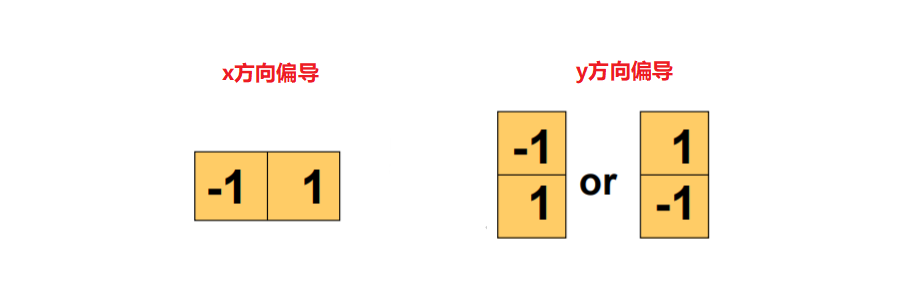对灰度图的x和y方向分别处理后的效果如下图：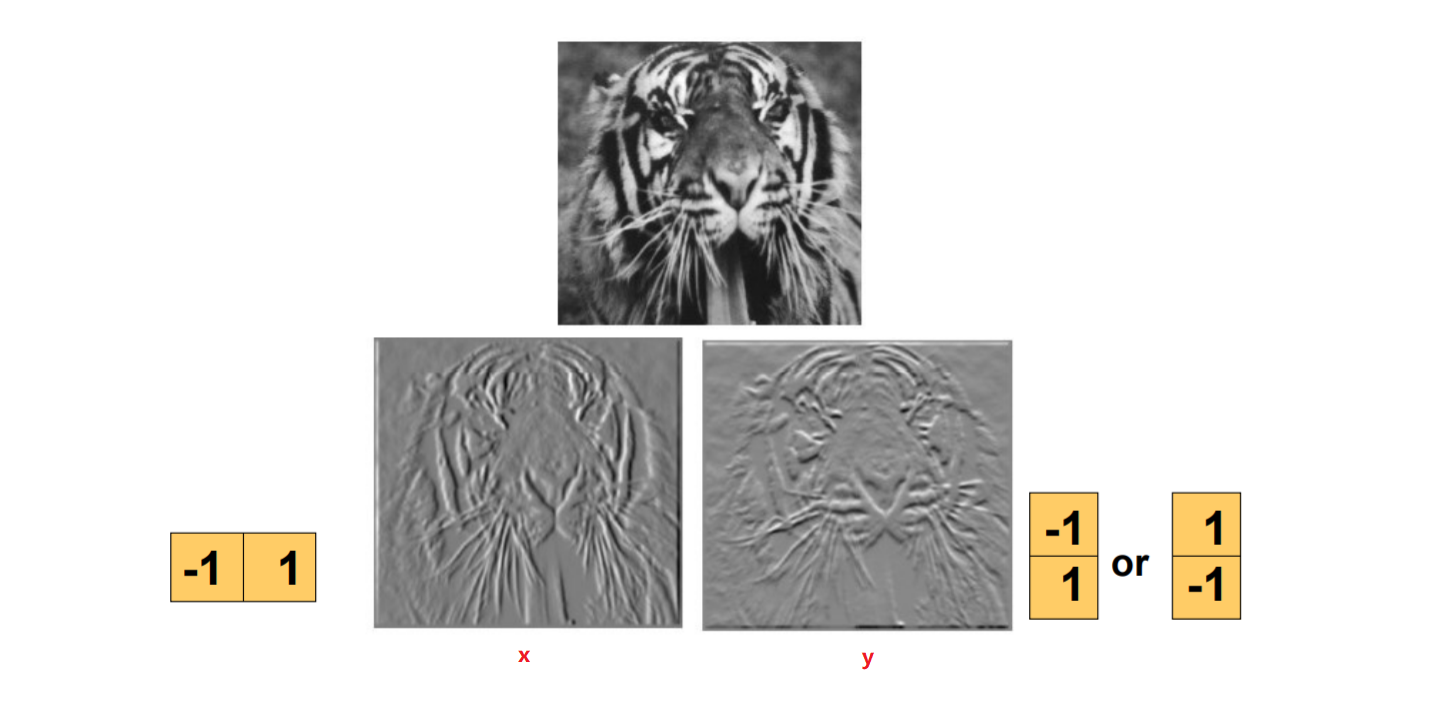• Roberts 算子 Roberts 算子是一种最简单的算子，是一种利用局部差分算子寻找边缘的算子。他采用对角线方向相邻两象素之差近似梯度幅值检测边缘。检测垂直边缘的效果好于斜向边缘，定位精度高，对噪声敏感，无法抑制噪声的影响。 1963年， Roberts 提出了这种寻找边缘的算子。 Roberts 边缘算子是一个 2x2 的模版，采用的是对角方向相邻的两个像素之差。 Roberts 算子的模板分为水平方向和垂直方向，如下所示，从其模板可以看出， Roberts 算子能较好的增强正负 45 度的图像边缘。
$$dx = \left[ \begin{matrix} -1 & 0\\ 0 & 1 \\ \end{matrix} \right]$$
$$dy = \left[ \begin{matrix} 0 & -1\\ 1 & 0 \\ \end{matrix} \right]$$
• Prewitt算子 Prewitt 算子是一种一阶微分算子的边缘检测，利用像素点上下、左右邻点的灰度差，在边缘处达到极值检测边缘，去掉部分伪边缘，对噪声具有平滑作用。Prewitt算子适合用来识别噪声较多、灰度渐变的图像。
$$dx = \left[ \begin{matrix} 1 & 0 & -1\\ 1 & 0 & -1\\ 1 & 0 & -1\\ \end{matrix} \right]$$
$$dy = \left[ \begin{matrix} -1 & -1 & -1\\ 0 & 0 & 0\\ 1 & 1 & 1\\ \end{matrix} \right]$$
• Sobel算子 Sobel算子是一种用于边缘检测的离散微分算子，它结合了高斯平滑和微分求导。Sobel 算子在 Prewitt 算子的基础上增加了权重的概念，认为相邻点的距离远近对当前像素点的影响是不同的，距离越近的像素点对应当前像素的影响越大，从而实现图像锐化并突出边缘轮廓。
$$dx = \left[ \begin{matrix} 1 & 0 & -1\\ 2 & 0 & -2\\ 1 & 0 & -1\\ \end{matrix} \right]$$
$$dy = \left[ \begin{matrix} -1 & -2 & -1\\ 0 & 0 & 0\\ 1 & 2 & 1\\ \end{matrix} \right]$$

## 图像梯度

$$\nabla f=[\frac{\partial f}{\partial x},\frac{\partial f}{\partial y}]$$

• 梯度指向强度增长最快的方向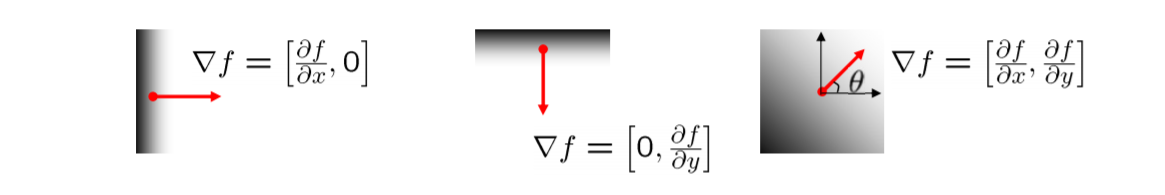• 梯度的角度 边的方向与梯度方向垂直 $$\theta = tan^{-1} (\frac{\partial f}{\partial y}/\frac{\partial f}{\partial x})$$

• 梯度的模长（幅值） 可以说明是边缘的可能性大小 $$||\nabla f|| = \sqrt{(\frac{\partial f}{\partial x})^2+(\frac{\partial f}{\partial y})^2}$$

• 处理图像后：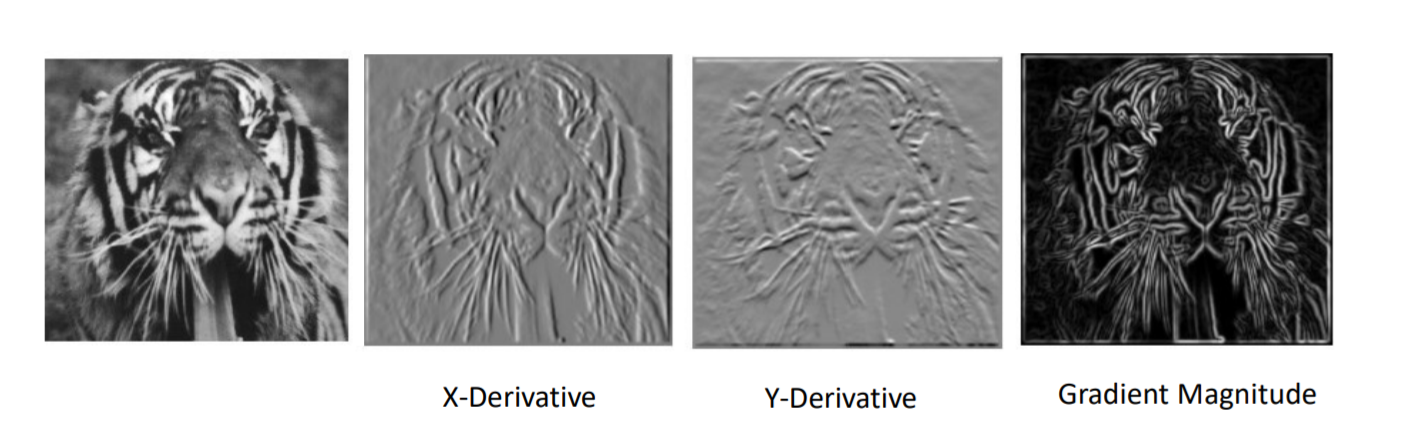## 高斯滤波器

1. 平滑处理：使用平滑滤波器去噪，使图像信号变得平滑

2. 再对处理后的信号求导，取极值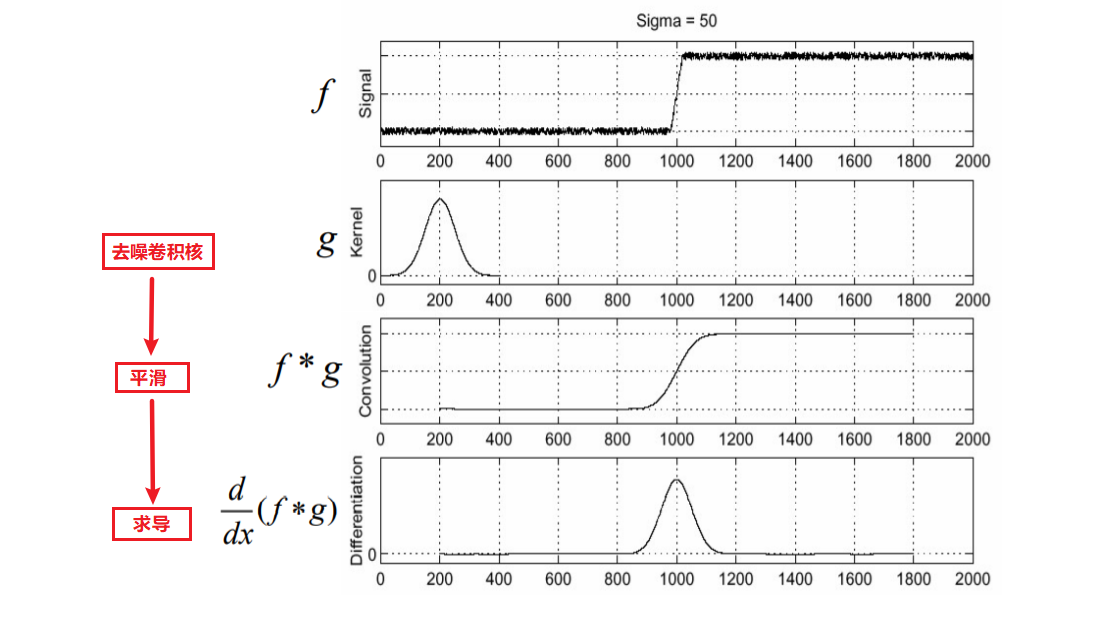3. 根据卷积的计算性质：$\frac{d}{dx}(f*g) = f * \frac{d}{dx}g$，先对平滑核求导，再进行卷积相乘来简化运算，减少运算量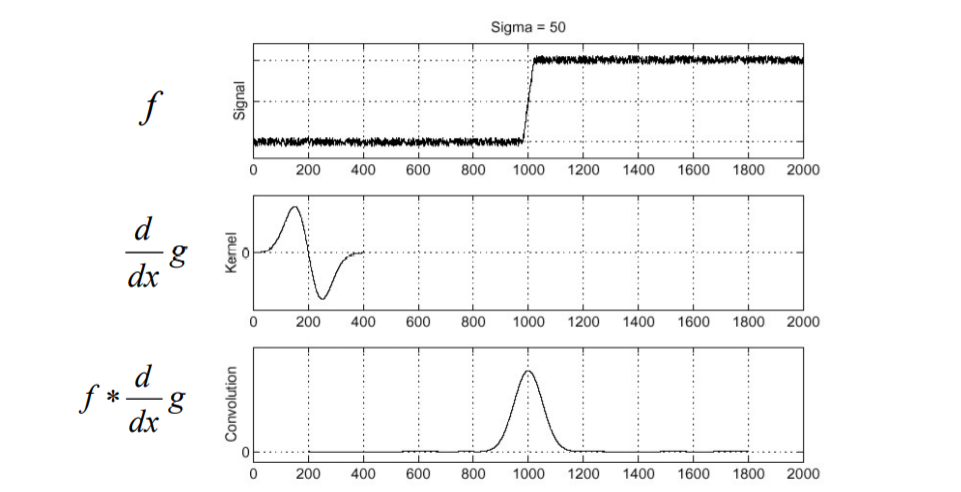## Canny 边缘检测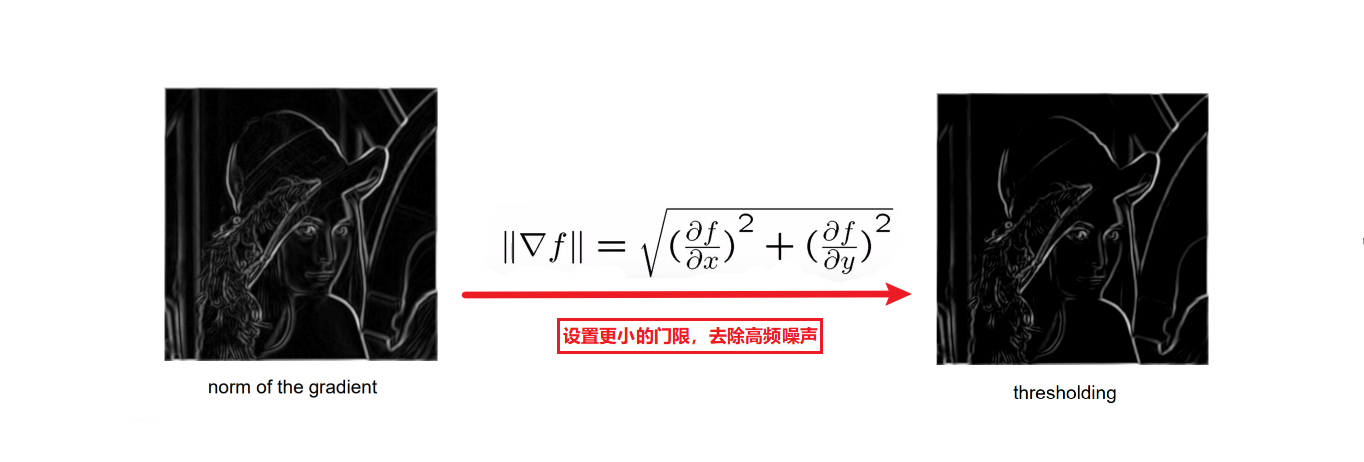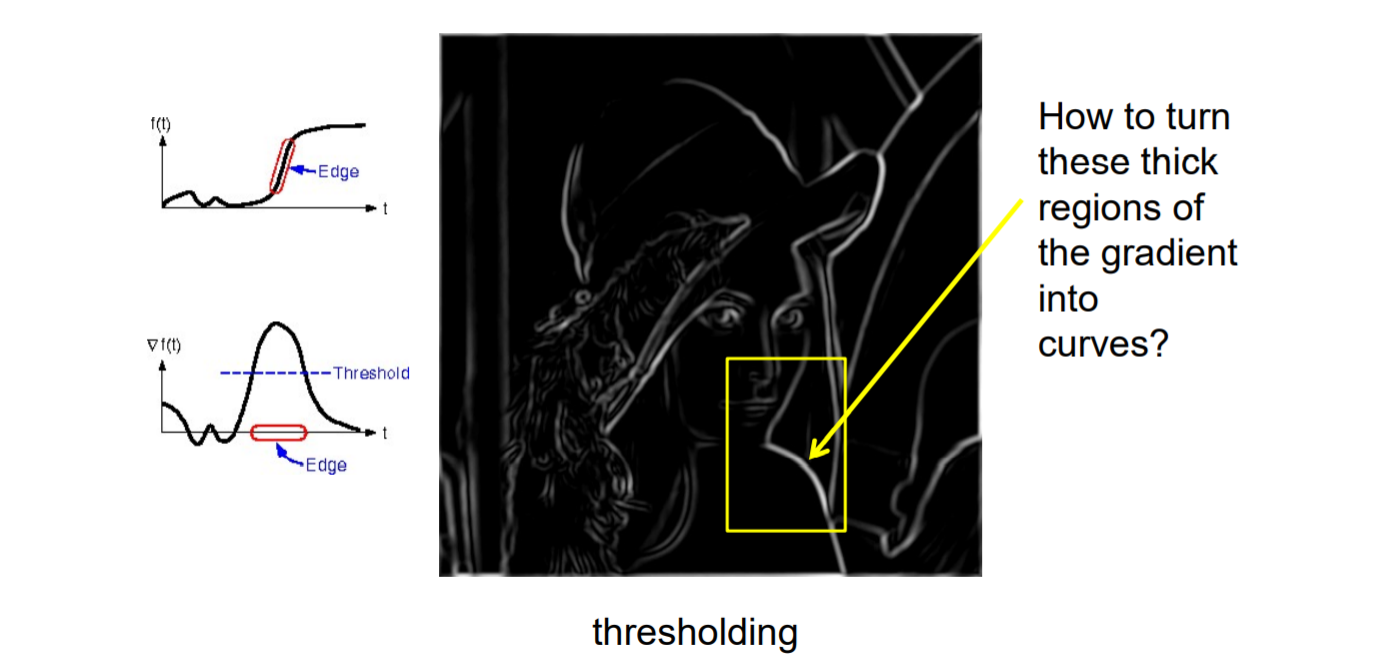方案

1. 检查像素是否沿梯度方向为局部最大值，选择沿边缘宽度的最大值作为边缘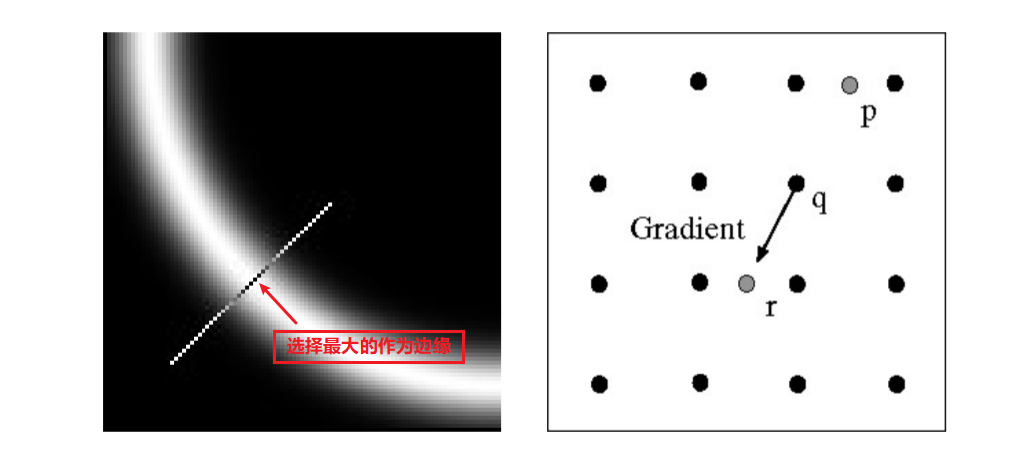2. 处理后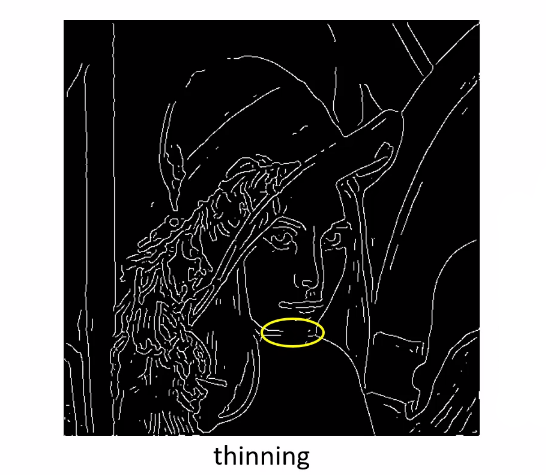经过上面的处理后，已经可以较为粗糙的得到图像的边缘图，但仍然存在问题，在有些部分的边 缘不连续，失去了很多信息如上图的 黄色区域 ，这是由于在门限化的过程中，设置过小，导致将需要的边缘滤除。

1. 先使用高门限将较粗的边检测出来，这些边都是比较鲁棒的，是噪声的可能性极低
2. 再降低门限，将较细的边显现出来
3. 将与高门限过滤出的边连接的低门限边保留，滤除没有连接的（不连续的）噪声1. 处理后可以得到更好的边缘效果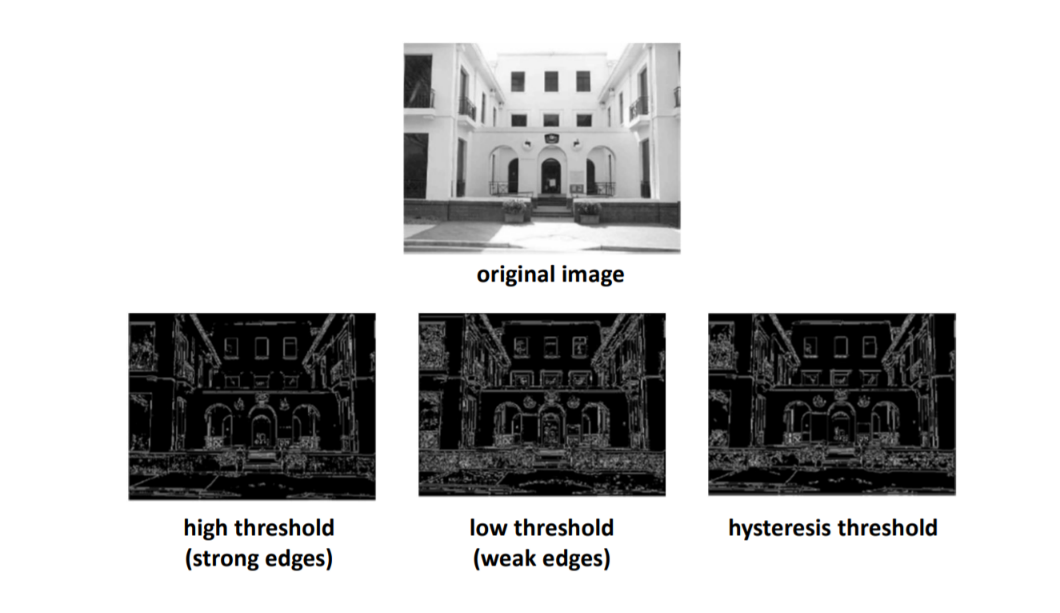##### Yasin Wang
###### Undergraduate Student of Artificial Intelligence

My interests include deep learning, artificial intelligence, computer vision and website development.